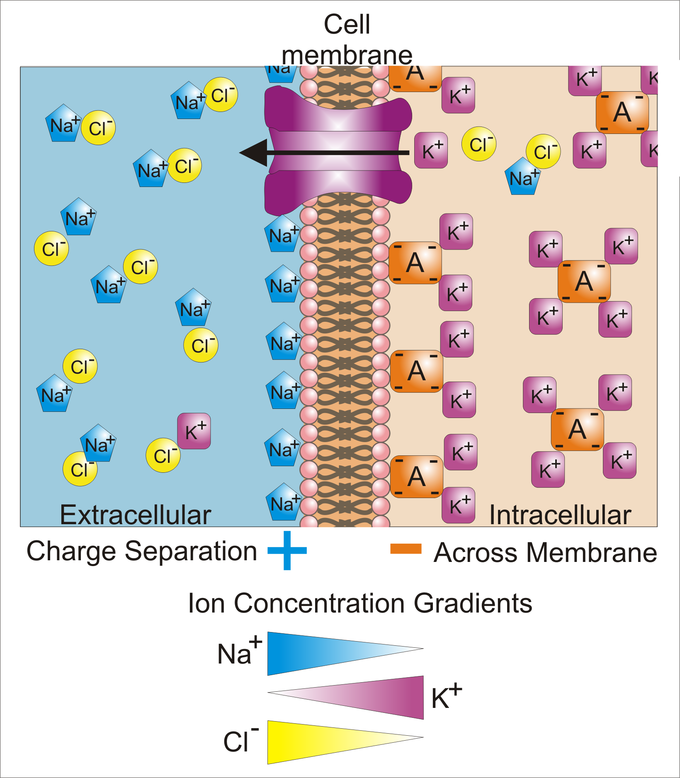10.5A: Principles of Electricity

When a neuron is stimulated, an electrical impulse is generated and conducted along the length of its axon. This process, called action potential, underlies many nervous system functions.

Learning Objectives

• Identify principles of electricity that are important in neurophysiology

Key Points

• Voltage and current are two important factors to consider in the study of neurons.
• The flow of electrical charge from one point to another is called current.
• Across the cellular membranes, potential difference is established between the outside of a cell and the inside of the cell which can affect the flow of current across the cell membrane.

Key Terms

• Voltage: The measure of potential energy generated by separated charge.
• current: The flow of electrical charge from one point to another.
• resistance: Hindrance to the flow of charge.

Voltage and current are two important factors to consider in the study of neurons. Voltage is the measure of potential energy generated by separated charge. It is measured in volts or millivolts. The greater the difference in charge between two points, the higher the voltage. Voltage is equal to the work that would have to be done per unit charge to move the charge between two points against a static electric field. Voltage may represent either a source of energy (electromotive force) or lost or stored energy (potential drop).

The flow of electrical charge from one point to another is called current. The amount of charge that moves between two points depends on two factors: voltage and resistance. Resistance is the hindrance to the flow of charge. Some substances with high resistance are insulators, like the myelin sheath.

In the body, electrical currents reflect the flow of ions across cell membranes. Since there is a slight difference in the number of positive and negative ions on the two sides of the cellular plasma membrane, there is a potential difference across the membranes. Diffusion arises from the statistical tendency of particles to redistribute from regions where they are highly concentrated to regions where the concentration is low. The difference in potential established between the outside of a cell and the inside of the cell can affect the flow of current across the cell membrane.Cellular Membrane: Differences in concentration of ions on opposite sides of a cellular membrane lead to a voltage called the membrane potential. Many ions have a concentration gradient across the membrane, including potassium (K+), which is at a high inside and a low concentration outside the membrane. Sodium (Na+) and chloride (Cl–) ions are at high concentrations in the extracellular region and low concentrations in the intracellular regions. These concentration gradients provide the potential energy to drive the formation of the membrane potential.

In electrically active tissue, the potential difference between any two points can be measured by inserting an electrode at each point and connecting both electrodes to to a specialized voltmeter.